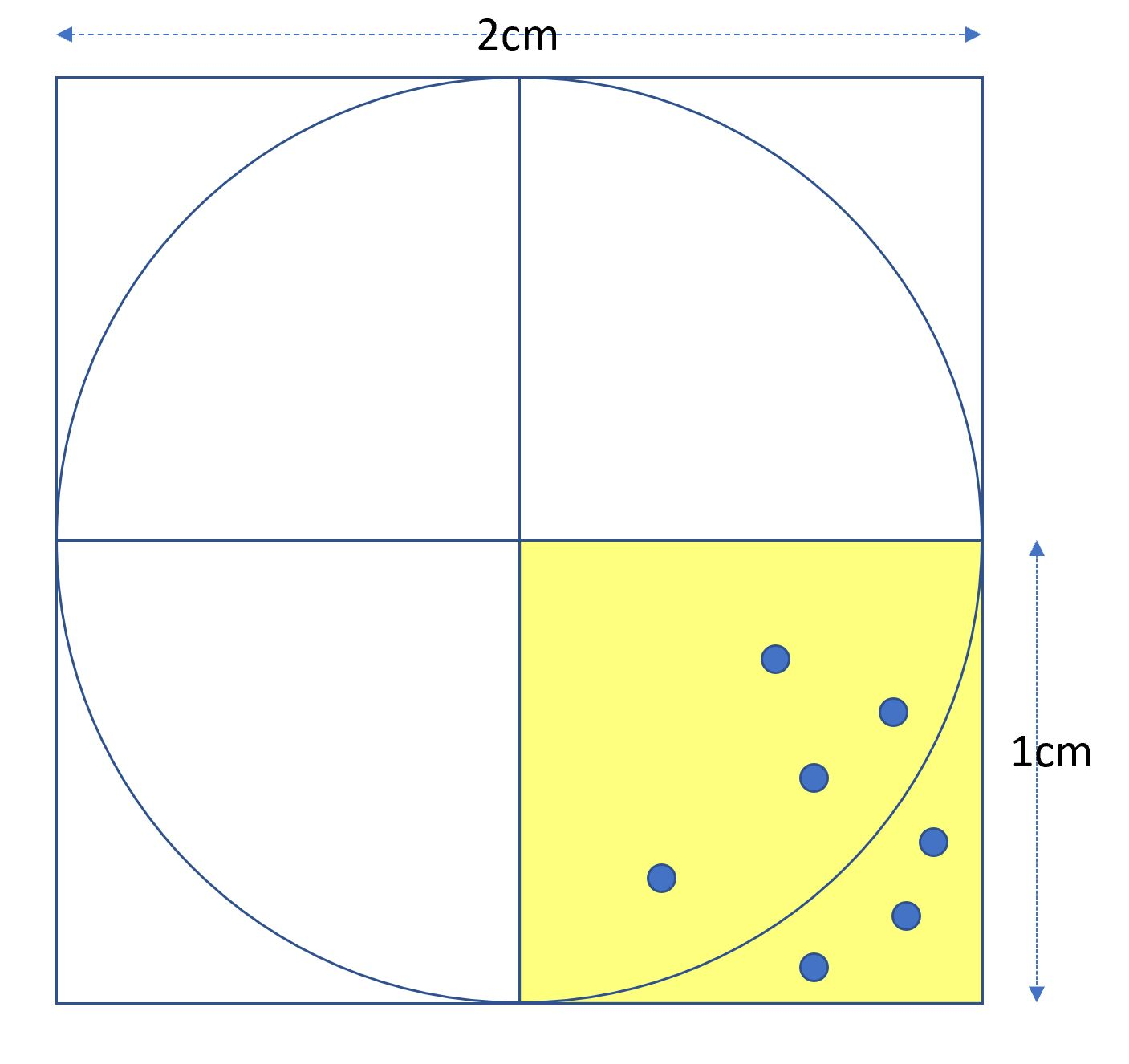# 原理#include <iostream>
#include <cmath.h>
#include <stdlib.h>
#include <time.h>
using namespace std;
bool circle(double x, double y){
double r=pow(pow(x,2)+pow(y,2), 0.5);
if (r<=1)return true;
else return false;
}
int main(){
srand(time(NULL));
unsigned long total=4000000000;
cout<<total<<endl;
unsigned int count=0;
for(int i=0;i<total;i++){
double x=rand()/(double)RAND_MAX;
double y=rand()/(double)RAND_MAX;
if (circle(x, y))count++;
}
double area=count/(double)total*4;
printf("%f",area);
}


4000000000
3.141522